Test: Chemical Kinetics - 3

# Test: Chemical Kinetics - 3

Test Description

## 30 Questions MCQ Test Physical Chemistry | Test: Chemical Kinetics - 3

Test: Chemical Kinetics - 3 for Chemistry 2022 is part of Physical Chemistry preparation. The Test: Chemical Kinetics - 3 questions and answers have been prepared according to the Chemistry exam syllabus.The Test: Chemical Kinetics - 3 MCQs are made for Chemistry 2022 Exam. Find important definitions, questions, notes, meanings, examples, exercises, MCQs and online tests for Test: Chemical Kinetics - 3 below.
Solutions of Test: Chemical Kinetics - 3 questions in English are available as part of our Physical Chemistry for Chemistry & Test: Chemical Kinetics - 3 solutions in Hindi for Physical Chemistry course. Download more important topics, notes, lectures and mock test series for Chemistry Exam by signing up for free. Attempt Test: Chemical Kinetics - 3 | 30 questions in 90 minutes | Mock test for Chemistry preparation | Free important questions MCQ to study Physical Chemistry for Chemistry Exam | Download free PDF with solutions
 1 Crore+ students have signed up on EduRev. Have you?
Test: Chemical Kinetics - 3 - Question 1

### The energy of activation of a forward reaction is 50Kcal. The energy of activation of a backward reaction is:

Test: Chemical Kinetics - 3 - Question 2

### The rate constant is numerically the same for three reactions of first, second and third orders respectively. When concentration is less than unity, the rate of reactions are, in order:

Test: Chemical Kinetics - 3 - Question 3

### The given graph represents: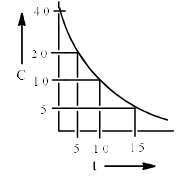Test: Chemical Kinetics - 3 - Question 4

Rate of a reaction. A + B → Product is given below as a function of different initial concentrations of A and B.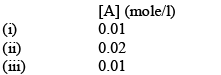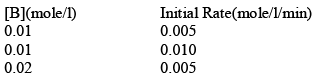The rate of the reaction is:

Test: Chemical Kinetics - 3 - Question 5

In a reaction: 2N2O5 → 4NO2 + O2 , the rate is expressed as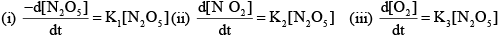The relation among K1, K2 and K3 is:

Detailed Solution for Test: Chemical Kinetics - 3 - Question 5

Explanation : As we know, for a reaction: 2N2O5 → 4NO2 + O2

=> -1/2 d[N2O5]/dt = 1/4 d[NO2]/dt = d[O2]/dt

So, 2k1 = k2 = 4k3

Test: Chemical Kinetics - 3 - Question 6

Calculate order of reaction A → product , from the following data: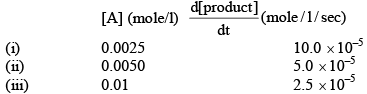Detailed Solution for Test: Chemical Kinetics - 3 - Question 6

By doubling the concentration the rate becomes half from (i) to (ii) also from (ii) to (iii).

Hence, B is correct.

Test: Chemical Kinetics - 3 - Question 7

A first order reaction is 50% complete in 30mins at 270C and in 10mins at 470C. At 270C, the activation energy of the reaction in KJ/mole is:

Detailed Solution for Test: Chemical Kinetics - 3 - Question 7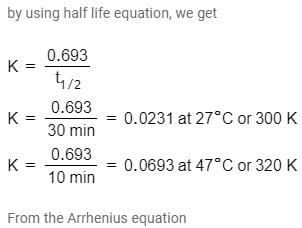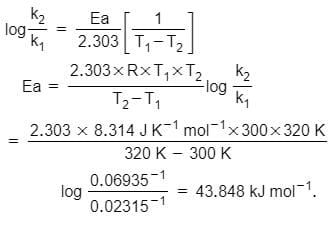Test: Chemical Kinetics - 3 - Question 8

What is the rate of simple reaction 2NO + O2 → 2 NO2 ; when the volume of the reaction vessel is doubled?

Test: Chemical Kinetics - 3 - Question 9

Which among the following procedures will lead to a change in rate constant ‘k’ of a reaction?

(I) A change in pressure
(II) Change in temperature
(III) Change in volume of the reaction vessel
(IV) An introduction of a catalyst

Test: Chemical Kinetics - 3 - Question 10

The concept of t1/2 is useful for the reaction of:

Test: Chemical Kinetics - 3 - Question 11

For a given set of reactions: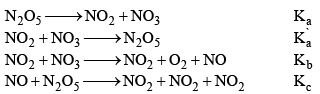The rate constant for the decay of N2O5 depends on:

Test: Chemical Kinetics - 3 - Question 12

The given reaction (pre-equilibrium mechanism)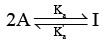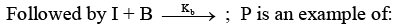Test: Chemical Kinetics - 3 - Question 13

The gas phase decomposition of NOBr is second order in [NOBr], with k = 0.810 M–1 s–1 at 100C. We start with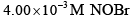in a flask at 100C. How many seconds does it take to use up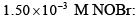2NOBr(g) → 2NO(g) + Br2(g)

Rate = k[NOBr]2

Test: Chemical Kinetics - 3 - Question 14

The concentration of a reactant undergoing decomposition was 0.1, 0.08 and 0.067 mol L–1 after 1.0, 2.0 and 3.0 hr respectively. The order of the reaction is:

Detailed Solution for Test: Chemical Kinetics - 3 - Question 14

Explanation : A ----->k P

-d[A]/dt = k[A](0 to n)

-{[A]0 - [A]}/(t2 - t1) = k[A](0 to n)

{[A] - [A]0}/(t2 - t1) = k[A](0 to n)

[A]0 = concentration at t1

and [A] = concentration at t2

{[A] - [A]0}/(t2 - t1) = k[A](0 to n)

(0.1-0.08)/(2-1) = k[0.1]n

k[0.1]n = 0.02......(1)

(0.08-0.067)/(3-2) = k[0.08]n

k[0.08]n = 0.013.........(2)

Dividing (1) by (2)

k[0.1]n/k[0.08]n = 0.02/0.013

{[0.1]/[0.08]}n = 1.5385

[1.25]n = 1.5385

[1.25]n = (1.25)2

n = 2

Test: Chemical Kinetics - 3 - Question 15

Plots showing the variation of the rate constant (k) with temperature (T) are given below. The plot that follows Arrhenius equation is:

Test: Chemical Kinetics - 3 - Question 16

A reaction of first-order completed 90% in 90 minutes, hence, it is completed 50% in approximately.

Test: Chemical Kinetics - 3 - Question 17

The unit of catalytic constant is equal to:

Test: Chemical Kinetics - 3 - Question 18

At 350K, the effective rate constant for a gaseous reaction A → P , which has a Lindeman-Hinshelwood mechanism are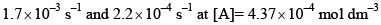and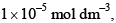respect ively. The rate constant for activation step in the mechanism is:

Test: Chemical Kinetics - 3 - Question 19

In the temperature range of 250 K to 450 K, the pre-exponent ial factor, A, for the reaction. Cl(g) + H2(g) → HCL(g) + H(g) is found to be equal to 1.20 x 1010 dm3 mol-1 s-1 . If M (Cl) = 35.453 g mol-1, M[H2] = 2.016 g mol-1, d(Cl) = 200 pm and d[H2] = 150 pm, the value of the Steric factor, P is:

Test: Chemical Kinetics - 3 - Question 20

The t1/2 of a reaction is doubled as the initial concentration of a reactant is increased 4 times. The order of the reaction is:

Detailed Solution for Test: Chemical Kinetics - 3 - Question 20

Explanation : A → P . For zero order reaction, rate= k. So, unit of k is

moles/sec.

- d[A]/dt = k

Upon integration between limits, [ A ] − [ A0 ] = − kt

At t1/2, [A]=[A0]/2.

Hence, t1/2 = [A0]/2k

Increased by 4 times, so it will become double

Test: Chemical Kinetics - 3 - Question 21

The relaxation time for the fast reaction: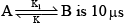and equilibrium constant is 1.0 x 10–3. The rate constant of the backward reaction is:

Detailed Solution for Test: Chemical Kinetics - 3 - Question 21

Explanation : Kb = (10 * 10-6) * 10-3

= 10-8 s-1

Test: Chemical Kinetics - 3 - Question 22

For which of the following reactions, the rate constant will be independent of ionic strength.

(I) H+ + Br + H2O2
(II) C2H5COOCH3 + OH
(III) S2O32– + I

Test: Chemical Kinetics - 3 - Question 23

Which of the following plots is correct for an ionic reaction:

Test: Chemical Kinetics - 3 - Question 24

For the first order isomerization of an organic compound at 1300C, the activation energy is 108.4 KJ mol–1 and the rate constant is 9.12 x 10–4 s–1. The standard entropy of activation for this reaction is:

Test: Chemical Kinetics - 3 - Question 25

For reaction system,

2NO(g) + O2 (g) → 2 NO2 (g)

Volume is suddenly reduced to half of its value by increasing the pressure on it. If the reaction is of first order with respect to O2 and second order with respect to NO, the rate of reaction will.

Test: Chemical Kinetics - 3 - Question 26

Rate = K[A][B]; If the volume of reaction vessel is suddenly reduced 1/4th of initial value. The new rate will be effected by:

Test: Chemical Kinetics - 3 - Question 27

Under the same reaction conditions, initial concentration of 1.386 mol dm–3 of a substance becomes half in 40 s and 20 s through first order and zero order kinetics, respectively., Ratio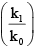of the rate constants for the first order k1 and zero order (k0) of the reaction is

Test: Chemical Kinetics - 3 - Question 28

T50 (Half-life period) of first-order reaction is 10 minute. Starting with 10 mol L–1. Rate after 20 minute is:

Test: Chemical Kinetics - 3 - Question 29

If the fermentation of sugar in an enzymatic solution that in 0.12 M. the concentration of the sugar is reduced to 0.06 M in 10 h and to 0.03 M in 20 h. What is the order of the reaction:

Test: Chemical Kinetics - 3 - Question 30

In a zero-order reaction for every 10° rise of temperature, the rate is doubled. If the temperature is increased from 10°C to 100°C, the rate of the reaction will become

## Physical Chemistry

67 videos|106 docs|31 tests
 Use Code STAYHOME200 and get INR 200 additional OFF Use Coupon Code
Information about Test: Chemical Kinetics - 3 Page
In this test you can find the Exam questions for Test: Chemical Kinetics - 3 solved & explained in the simplest way possible. Besides giving Questions and answers for Test: Chemical Kinetics - 3, EduRev gives you an ample number of Online tests for practice

## Physical Chemistry

67 videos|106 docs|31 tests